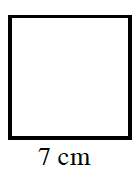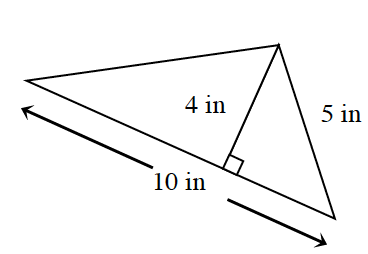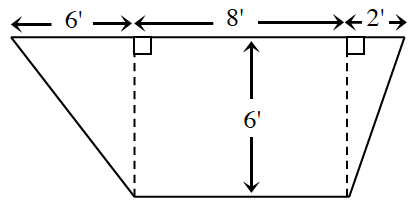### Home > GB8I > Chapter 5 Unit 6 > Lesson INT1: 5.3.2 > Problem5-113

5-113.

Calculate the area of each figure below. Show all work. Remember to include units in your answer. Homework Help ✎

1. a square:$49$ square cm

1.1.$\frac{1}{2}(b_{1}+b{_{2}})h$

$\frac{1}{2}(16+8)6\text{ feet}^2$